# 基于贝叶斯网络的无人机任务可靠性建模方法The Reliability Modeling Method of UAV Mis-sion Based on Bayesian Network

DOI: 10.12677/CSA.2020.109167, PDF, HTML, XML, 下载: 95  浏览: 214

Abstract: Due to its inherent dexterity, UAV is not easy to be detected when carrying out activities, and it can often achieve unexpected effects. Even if it is detected and destroyed, the loss will be much less than the loss of human aircraft. Therefore, there is bound to be a place for UAV in future wars. At the same time, the UAV mission reliability is related to the success of the task. In order to explore the reliability of the UAV mission modeling method, this article is based on Bayesian network is studied. First of all, according to the topology of the unmanned aerial vehicle (UAV) each subsystem mission reliability block diagram is established, and on the basis of mission reliability diagram coverted into Bayesian networks. Finally, the feasibility of model is validated by an example. The research in this paper provides support for the follow-up research on the reliability of UAV mission.

1. 引言

2. 无人机系统Table 1. Influence parameters of abnormal and extreme environmental factors

3. 无人机任务可靠性分析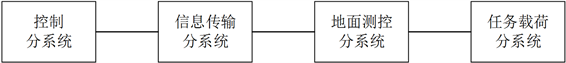Figure 1. Structure block diagram of UAV system

3.1. 控制分系统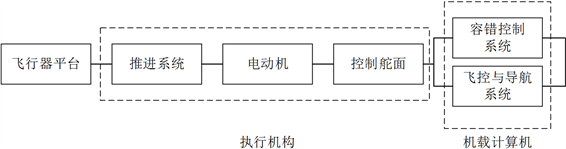Figure 2. Structure block diagram of a typical UAV control subsystem

${P}_{ti}=1-{\text{e}}^{-\lambda it}$ (1)

${P}_{ti}={k}_{a}\cdot {k}_{b}\cdot {k}_{m}\left(1-{\text{e}}^{-\lambda it}\right)$ (2)

3.2. 信息传输分系统

${P}_{ri}={k}_{a}\cdot {k}_{b}\cdot {k}_{m}\cdot \frac{MTBF}{MTBF+MTTR}$ (3)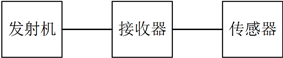Figure 3. Structure diagram of information transmission subsystem

3.3. 地面测控分系统

3.4. 任务载荷分系统

3.5. 无人机任务可靠性框图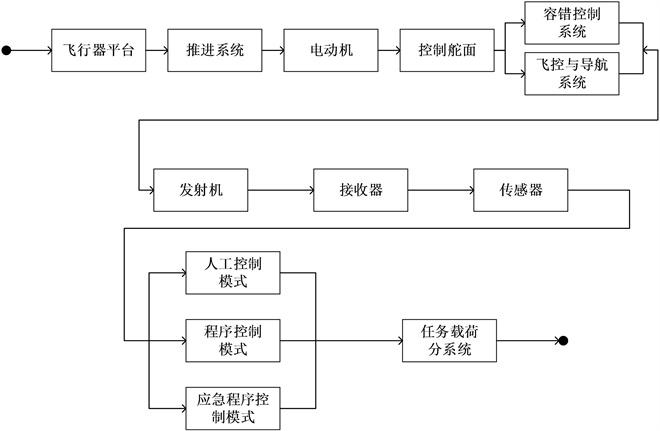Figure 4. Single UAV mission reliability block diagram

${P}_{i}=\underset{i=1}{\overset{4}{\prod }}{P}_{ti}\cdot \left(1-\left(1-{P}_{t4}\right)\left(1-{P}_{t5}\right)\right)\cdot \underset{i=1}{\overset{3}{\prod }}{P}_{ri}\cdot \left(1-\underset{i=1}{\overset{3}{\prod }}\left(1-{P}_{ki}\right)\right)\cdot {P}_{n}$ (4)

4. 贝叶斯网络模型

4.1. 贝叶斯网络基本理论

4.2. 无人机任务可靠性的贝叶斯网络建模过程

4.2.1. 贝叶斯网络的建立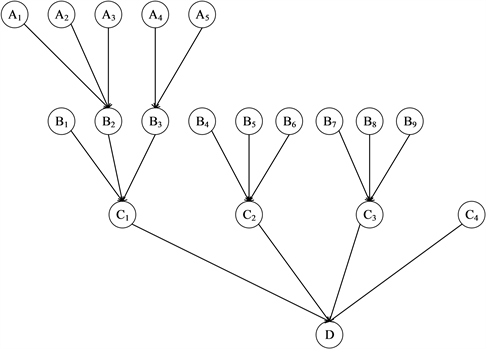Figure 5. Bayesian network model of UAV

4.2.2. 贝叶斯网络推理

$P\left(D\right)=\underset{i=1}{\overset{4}{\prod }}p\left({C}_{i}\right)$ (5)

C1和C2分别由B1、B2、B3和B4、B5、B6串联组成，C3由B7、B8、B9并联组成可得：

$p\left({C}_{1}\right)=p\left({B}_{1}\right)\cdot p\left({B}_{2}\right)\cdot p\left({B}_{3}\right)$ (6)

$p\left({C}_{2}\right)=p\left({B}_{4}\right)\cdot p\left({B}_{5}\right)\cdot p\left({B}_{6}\right)$ (7)

$p\left({C}_{3}\right)=1-\left(1-p\left({B}_{7}\right)\right)\cdot \left(1-p\left({B}_{8}\right)\right)\cdot \left(1-p\left({B}_{9}\right)\right)$ (8)

$p\left({B}_{2}\right)=p\left({A}_{1}\right)\cdot p\left({A}_{2}\right)\cdot p\left({A}_{3}\right)$ (9)

$p\left({B}_{3}\right)=1-\left(1-p\left({A}_{4}\right)\right)\cdot \left(1-p\left({A}_{6}\right)\right)$ (10)

5. 建模与计算Table 2. Failure probability of the original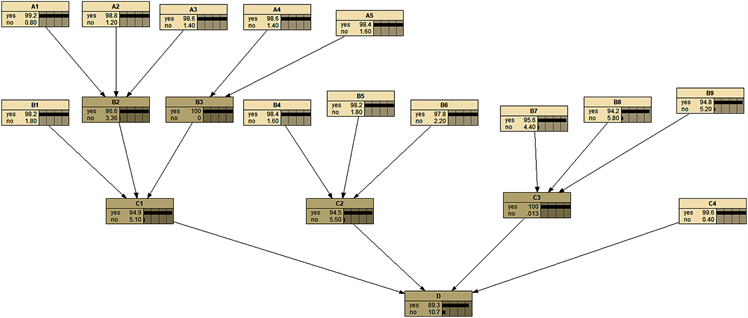Figure 6. Mission reliability of UAV

6. 结束语

  金国栋, 卢利斌, 王剑锋. 无人侦察机任务可靠性评估模型[J]. 战术导弹技术, 2005(2): 27-30+51.  翟胜. 基于贝叶斯网络的复杂系统可靠分析方法研究与应用[D]: [博士学位论文]. 天津: 天津工业大学, 2015.  Camille Alain Rabbath, Nicolas Lechevin , 拉巴特, 等. 协同无人机系统安全性与可靠性[M]. 北京: 国防工业出版社, 2015.  昂海松. 无人机系统概念和关键技术[J]. 无人系统技术, 2018, 1(1): 66-71.